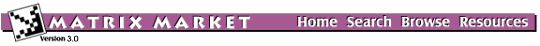## MVMTLS: Tolosa Matrix

### from the NEP Collection

Matrix Generator MVMTLS
Source: S. Godet-Thobie, CERFACS and C. Bès, Aerospatiale, France
Discipline: Aeroelasticity
Language: Fortran
Output format: matrix-vector multiply

The Tolosa matrix arises in the stability analysis of a model of an airplane in flight. The interesting modes of this system are described by complex eignevalues whose imaginary parts lie in a prescribed frequency range. The task is to compute the eigenvalues with largest imaginary parts. The problem has been analyzed at CERFACS (Centre European de Recherche et de Formation Avancee en Calcul Scientifique) in cooperation with the Aerospatiale Aircraft division.

The matrix is a sparse 5 x 5 block matrix of order n = 90 + 5k. In practice, k is around 104. When n = 90, each block is of dimension 18 x 18 and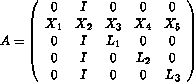where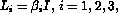and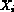and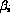are given data. In general,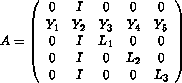where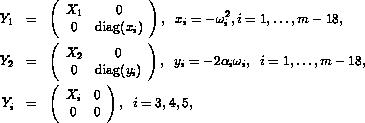and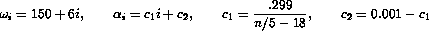The following figures show the eigenvalue distribution of Tolosa matrices of orders 90 and 340.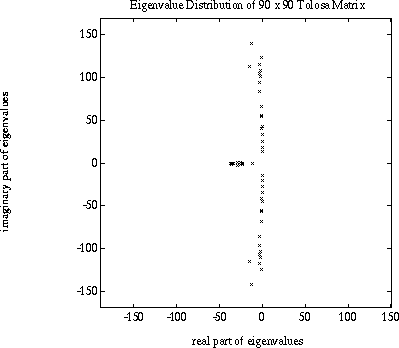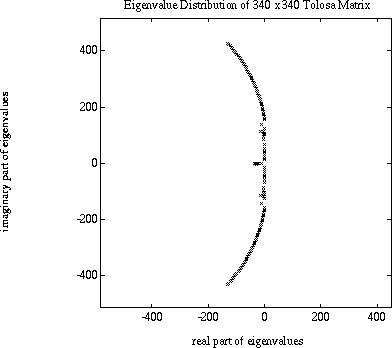Parameters:

 N order of the matrix. Must be 90+5K for some nonnegative integer K.